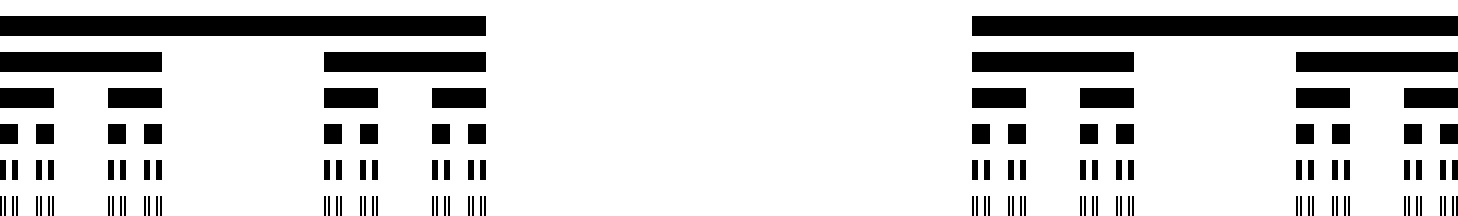Here we have collected our favourite sets, from the Mandelbrot set to the Mahut-Isner set!A set is a collection of items. Sprinkled throughout Issue 04 of Chalkdust were some of our favourite sets. Here we have collected them together and we’d really love to hear yours. You can write about them at the bottom of this post!

### Mandelbrot set (Andrea Bertozzi)

During our interview with Andrea Bertozzi she shared with us why the Mandelbrot set is her favourite set: “My research group developed an efficient algorithm for tracking the boundaries of sets on different scales. I thought we could apply it to fractals so we demonstrated it on the Mandelbrot set.”The figure above is the Mandelbrot set computed on a 100 $\times$ 100 grid and the figure refines the grid all the way up to 1000 $\times$ 1000 pixels.

### Cantor set (Belgin Seymenoğlu)

If you want to construct my favourite set, start with the interval $[0, 1]$. Next, remove the open middle third interval. This gives you two line segments: $[0, 1/3]$ and $[2/3, 1]$. Again, delete the middle third for each remaining interval (which leaves you with four new intervals). Now repeat the final step ad infinitum.Once you’re done, you’re left with the Cantor set (also called the Cantor comb). But what does the Cantor set look like? Infinitely many discrete points? An infinite collection of line segments? The answer is a bit of both, and that’s because the dimension of my favourite set is neither zero nor one: it’s $\ln2/\ln3\approx 0.63093$. The other wonderful feature of this set is that it’s a fractal, so if you zoom in on a tiny portion, you get the Cantor set again!

### The empty set (Rob Beckett)

My favourite set is the empty set ($\varnothing$). The empty set is the only set of its kind and contains no elements. The empty set is a subset of any subset but the only subset of the empty set is itself.The empty set is not nothing, but is a set that contains nothing. If I had a bag with multicoloured counters in, these are the elements of a non-empty set. On the other hand, if I had a bag with no counters in, there are no elements in the set and this is an example of the empty set.

### Numbers (Matthew Wright)

My favourite set is:

$$\hspace{-2mm}\{\{\},\{\{\}\},\{\{\},\{\{\}\}\},\{\{\},\{\{\}\},\{\{\},\{\{\}\}\}\},\{\{\},\{\{\}\},\{\{\},\{\{\}\}\},\{\{\},\{\{\}\},\{\{\},\{\{\}\}\}\}\}$$

or in other words, the number 5. In set theory, numbers are constructed as follows:

Zero is defined to be the empty set: $0 = \{\} = \varnothing$; one is the set containing the empty set: $1 = \{\{\}\}=\{\varnothing\}$; and the number $n$ is defined as the set containing all the previous numbers: $n = \{0,1,…..,n-1\}.$

As you can see, this definition causes things to get complicated quite quickly!

### $\aleph_1$ (Matthew Scroggs)

If you could count forever, you would reach infinity. You might be surprised to learn that there are bigger things than this infinity. My favourite set, $\aleph_1$ (aleph one), is the smallest set that is bigger than the counting infinity.

Cantor’s diagonal argument (if you’ve not heard of it, Google it!) can be used to show that there are more real numbers than natural numbers, and therefore that one infinite thing is bigger than another.

But $\aleph_1$ is even weirder than this. It is not known whether or not $\aleph_1$ and the real numbers are the same size. In fact, this is not just unknown, but it cannot be proven either way using the standard axioms of set theory! (The suggestion that they are the same size is called the continuum hypothesis.) So my favourite set is bigger than the smallest infinity—but we can’t work out by how much.

### Mahut-Isner set (Mattheas Recht)

My favourite set is the final set in the match between Nicolas Mahut and John Isner at Wimbledon in 2010. The set lasted over 11 hours with a final score of 70–68 to Isner.